# Important Questions for Class 12 Physics Chapter 10 Wave Optics## myCBSEguide App

Complete Guide for CBSE Students

NCERT Solutions, NCERT Exemplars, Revison Notes, Free Videos, CBSE Papers, MCQ Tests & more.

Important Questions for Class 12 Physics Chapter 10 Wave Optics. We know Physics is tough subject within the consortium of science subjects physics is an important subject. But if you want to make career in these fields like IT Consultant, Lab Technician, Laser Engineer, Optical Engineer etc. You need to have strong fundamentals in physics to crack the exam. myCBSEguide has just released Chapter Wise Question Answers for class 12 Physics. There chapter wise Extra Questions with complete solutions are available for download in myCBSEguide website and mobile app. These Questions with solution are prepared by our team of expert teachers who are teaching grade in CBSE schools for years. There are around 4-5 set of solved Physics Extra Questions from each and every chapter. The students will not miss any concept in these Chapter wise question that are specially designed to tackle Board Exam. We have taken care of every single concept given in CBSE Class 12 Physics syllabus and questions are framed as per the latest marking scheme and blue print issued by CBSE for class 12.

Class 12 Physics Extra Questions

## CBSE Class 12 Physics Chapter 10 Extra Questions

Class – 12 Physics (Wave Optics)

1. Referring to the Young’s double slit experiment, if D is the distance from two slit plane to screen and d the distance between two coherent sources then fringe width is given by

1. {tex}\frac{{3\lambda D}}{d}{/tex}
2. {tex}\frac{{2 \lambda D}}{d}{/tex}
3. {tex}\frac{{\lambda D}}{d}{/tex}
4. {tex}\frac{{\lambda D}}{2d}{/tex}
2. Monochromatic light of wavelength 589 nm is incident from air on a water surface. What is the wavelength and speed of reflected light?
1. 589 nm, 3 {tex} \times {\rm{ 1}}{0^{\rm{8}}}{/tex} m/s
2. 599 nm, {tex}{\rm{3 }} \times {\rm{ 1}}{0^{\rm{8}}}{/tex}m/s
3. 582 nm, {tex} \times {\rm{ 1}}{0^{\rm{8}}}{/tex} m/s
4. 590 nm, 3 {tex} \times {\rm{ 1}}{0^{\rm{8}}}{/tex} m/s
3. Referring to the Young’s double slit experiment, Phase difference corresponding to a Path Difference of {tex}\frac{\lambda }{3}{/tex} is

1. {tex}{\rm{18}}0^\circ {/tex}
2. {tex}{\rm{9}}0^\circ {/tex}
3. {tex}{\rm{6}}0^\circ {/tex}
4. {tex}{\rm{12}}0^\circ {/tex}
4. Light of wavelength 5000 Å falls on a plane reflecting surface. What are the wavelength and frequency of the reflected light? For what angle of incidence is the reflected ray normal to the incident ray?

1. {tex}{\rm{53}}00{\rm{ }}\mathop A\limits^0,{\rm{ 6 }} \times {\rm{ 1}}{0^{{\rm{14}}}}{\rm{Hz}};{\rm{ 65}}^\circ {/tex}
2. {tex}{\rm{5}}000{\rm{ }}\mathop A\limits^0,{\rm{ 6 }} \times {\rm{ 1}}{0^{{\rm{14}}}}{\rm{Hz}};{\rm{ 45}}^\circ {/tex}
3. {tex}{\rm{52}}00{\rm{ }}\mathop A\limits^0,{\rm{ 6 }} \times {\rm{ 1}}{0^{{\rm{14}}}}{\rm{Hz}};{\rm{ 55}}^\circ {/tex}
4. {tex}{\rm{54}}00{\rm{ }}\mathop A\limits^0,{\rm{ 6 }} \times {\rm{ 1}}{0^{{\rm{14}}}}{\rm{Hz}};{\rm{ 5}}0^\circ {/tex}
5. In a double-slit experiment the angular width of a fringe is found to be 0.2° on a screen placed 1 m away. The wavelength of light used is 600 nm. What will be the angular width of the fringe if the entire experimental apparatus is immersed in water? Take refractive index of water to be {tex}\frac{4}{3}{/tex}.

1. 0.12°
2. 0.15°
3. 0.14°
4. 0.13°
6. When light travels from a rarer to a denser medium, the speed decreases. Does this decrease in speed imply a decrease in the energy carried by the light wave? Justify your answer.

7. Two monochromatic waves emanating from two coherent sources have the displacements represented by,
{tex}y _ { 1 } = a \cos \omega t{/tex}
and {tex}y _ { 2 } = a \cos ( \omega t + \phi ){/tex},
where, {tex}\phi{/tex} is the phase difference between the two waves. Show that the resultant intensity at a point due to their superposition is given by
{tex}I = 4 I _ { 0 } \cos ^ { 2 } \phi / 2{/tex}, where {tex}I_0 = a^2.{/tex}

8. Why are coherent sources required to create interference of light?

9. In Young’s double slit experiment, monochromatic light of wavelength 600 nm illuminates the pair of slits and produces an interference pattern in which two consecutive bright fringes are separated by 10 mm. Another source of monochromatic light produces the interference pattern in which the two consecutive bright fringes are separated by 8 mm. Find the wavelength of light from the second source. What is the effect on the interference fringes if the monochromatic source is replaced by a source of white light?

10. Define the term linearly polarised light. When does the intensity of transmitted light become maximum, if a polaroid sheet rotated between two crossed polaroids?

11. Two towers on top of two hills are 40 km apart.The line joining them passes 50 m above a hill halfway between the towers. What is the longest wavelength of radio waves, which can be sent between the towers without appreciable diffraction effects?

12. When one of the slits in Young’s experiment is covered with a transparent sheet of thickness {tex}3.6 \times {10^{ – 3}}cm{/tex} the central fringe shifts to a position originally occupied by the 30th bright fringe. If {tex}\lambda = 6000\mathop {\text{A}}\limits^{\text{o}}{/tex} , find the refractive index of the sheet.

13. Use Huygens’ principle to show how a plane wavefront propagates from a denser to rarer medium. Hence, verify Snell’s law of refraction.

1. Unpolarised light of intensity I0 passes through two Polaroids P1 and P2 such that pass axis of P2 makes an angle {tex}\theta{/tex} with the pass axis of P1. Plot a graph showing the variation of intensity of light transmitted through P2 as the angle {tex}\theta {/tex} varies from 0° to 180°.
2. A third Polaroid P3 is placed between P1 and P2 with pass axis of P3 making an angle {tex}\beta {/tex} with that of P1 and the angle between P1 and P2 is {tex}\theta {/tex}. If I1, I2 and I3 represent the intensities of light transmitted by P1, P2 and P3, then determine the values of angle {tex}\theta {/tex} and I3 for which I1 = I2 = I3.
1. In a double slit experiment using the light of wavelength 600 nm, the angular width of the fringe formed on a distant screen is 0.1°. Find the spacing between the two slits.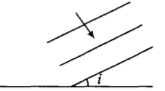2. Light of wavelength 500 {tex}\mathop {\text{A}}\limits^{\text{o}} {/tex} propagating in air gets partly reflected from the surface of water. How will the wavelengths and frequencies of the reflected and refracted light be affected?

Class – 12 Physics (Wave Optics)

1. {tex}\frac{{\lambda D}}{d}{/tex}
Explanation: {tex}\beta = \frac{{\lambda D}}{d}{/tex}
where lambda is the wavelength of light
Dis the distance of coherent sources from screen
d is the distance between the slits
1. 589 nm, 3 {tex} \times {\rm{ 1}}{0^{\rm{8}}}{/tex} m/s
Explanation: There is no change in speed of light and wave length when a wave gets reflected from a given surface.
1. 120o
Explanation: Phase difference = {tex}\frac{2\pi}{\lambda}{/tex} (path difference)
{tex} \Rightarrow \; \frac{2\pi }{\lambda} \times\frac{\lambda }{3} = \frac{{2\pi }}{3}=\frac{2\times180^\circ}{3}=120^\circ{/tex}
1. {tex} {\rm{5}}000{\rm{ }}\mathop A\limits^0,{\rm{ 6 }} \times {\rm{ 1}}{0^{{\rm{14}}}}{\rm{Hz}};{\rm{ 45}}^\circ {/tex}
Explanation: There is no change in wavelength of light in reflection.
use {tex}v=\nu\lambda{/tex}
1. 0.15°
Explanation: use {tex}\theta 1 = \frac{\theta }{\mu }{/tex}
{tex}{\theta 1 = \frac{{0.2}}{{1.33}} = .15}{/tex}
1. When light travels from a rarer to denser medium, its frequency remains unchanged. It is because, frequency is an inherent property of light.
We know that , {tex}E=h\nu{/tex}
As the speed decreases wavelength also decreases . So the frequency remains same.
Hence, {tex}h \ and  \ \nu{/tex} both are constant.
Energy remains unchanged.
Speed decreases due to decrease in wavelength of wave but energy carried by the light wave depends on the amplitude of the wave.
2. Given,
{tex}y _ { 1 } = a \cos \omega t{/tex}
{tex}y _ { 2 } = a \cos ( \omega t + \phi ){/tex}
The resultant displacement is given by
{tex}y = y_1 +y_2{/tex}
{tex}= a \cos \omega t + a \cos ( \omega t + \phi ){/tex}
{tex}= a \cos \omega t + a \cos \omega t \cos \phi – a \sin \omega t \sin \phi{/tex}
{tex}= a \cos \omega t ( 1 + \cos \phi ) – a \sin \omega t \sin \phi{/tex}
Put {tex}R \cos \theta = a ( 1 + \cos \phi ){/tex} …… (i)
{tex}R \sin \theta = a \sin \phi{/tex} ……. (ii)
By squaring and adding Eqs. (i) and (ii), we get
{tex}R ^ { 2 } = a ^ { 2 } \left( 1 + \cos ^ { 2 } \phi + 2 \cos \phi \right) + a ^ { 2 } \sin ^ { 2 } \phi{/tex}
{tex}= 2 a ^ { 2 } ( 1 + \cos \phi ) = 4 a ^ { 2 } \cos ^ { 2 } \frac { \phi } { 2 }{/tex}
{tex}\therefore \quad I = R ^ { 2 } = 4 a ^ { 2 } \cos ^ { 2 } \frac { \phi } { 2 } {/tex}
{tex}\therefore I = 4 I _ { 0 } \cos ^ { 2 } \frac { \phi } { 2 }{/tex}
3. To observe interference fringe pattern, there is need to have coherent sources of light which can produce light of constant phase difference or a zero phase difference (Which is a prior condition for creation of interference).
4. Here, we are given young’s double slit experiment.
Wavelength of monochromatic light, {tex}\lambda_1 = 600 nm = 600 \times 10^{-9}m{/tex}
Fringe width, {tex}\beta _1=10mm=10^{-2}m{/tex}
Fringe width,{tex}\beta _2=8mm=8 \times 10^{-3}m{/tex}
Wavelength of light from the second source=?
Fringe width due to first source , {tex}\beta_1 ={\lambda_1D \over d}{/tex}
Fringe width due to second source , {tex}\beta_2 ={\lambda_2D \over d}{/tex}
{tex}\lambda _ { 2 } = \frac { \beta _ { 2 } } { \beta _ { 1 } } \times \lambda _ { 1 } = \frac { 8 \times 10 ^ { – 3 } } { 10 \times 10 ^ { – 3 } } \times 600 \times 10 ^ { – 9 }{/tex}
{tex}\lambda _ { 2 } = 480 \times 10 ^ { – 9 } \mathrm { m }{/tex}
{tex}\Rightarrow \quad \lambda _ { 2 } = 480 \mathrm { nm }{/tex}
5. Linearly polarised light is the light wave in which the vibration of electric field vectors are confined in one plane and parallel to one unique direction by passing an unpolarised light through a polariser.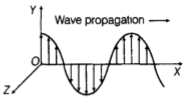The intensity of transmitted light becomes maximum when the inserted polaroid and analyser (the second polaroid which receives light that is transmitted by the first polaroid and analyse whether the light is polarised or not) have their axes parallel to each other. i.e. the angle of rotation when becomes zero degree or π radian between the polariser and analyser.
6. Distance of aperture from tower ZF {tex} = \frac{{40}}{2} = 20km = 20 \times {10^3}m{/tex}
Fresnel distance, {tex}{Z_F} = \frac{{{a^2}}}{\lambda }{/tex}
{tex} \Rightarrow \lambda = \frac{{{a^2}}}{{{Z_F}}} = \frac{{{{\left( {50} \right)}^2}}}{{20 \times {{10}^3}}}{/tex}
{tex}\lambda = 125 \times {10^{ – 3}}m = 12.5cm{/tex}
7. The position of the 30th bright fringe is given by
{tex}{x_n} = n\frac{{\lambda D}}{d}{/tex}
{tex}{x_{30}} = 30\frac{{\lambda D}}{d}{/tex}
Hence the shift of the central fringe is
{tex}x_{30} = 30\frac{{\lambda D}}{d}{/tex}
But {tex}x_{30} = \frac{D}{d}(\mu – 1)t{/tex}
{tex}\therefore 30\frac{{\lambda D}}{d} = \frac{D}{d}(\mu – 1)t{/tex}
{tex}\Rightarrow (\mu – 1) = \frac{{30\lambda }}{t}{/tex}{tex} = \frac{{30 \times (6000 \times {{10}^{ – 10}})}}{{(3.6 \times {{10}^{ – 5}})}} = 0.5{/tex}
or {tex}\mu = 1.5{/tex}
8. According to Huygens’ principle, Every point on a wave-front may be considered a source of secondary spherical wavelets which spread out in the forward direction at the speed of light. The new wave-front is the tangential surface to all of these secondary wavelets.
A surface touching these secondary wavelets, tangentially in the forward direction at any instant gives the new wavefront at that instant. This is called secondary wavefront.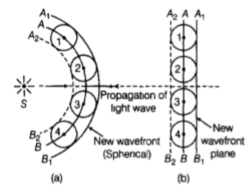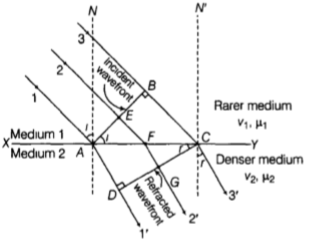If v1, v2 are the speeds of light into two mediums 1 and 2 respectively and t is the time taken by light to go from B to C or A to D or E to G through F, then from {tex}\Delta A F E , \sin i = E F / A F{/tex}
In {tex}\Delta F G C , \sin r = F G / F C {/tex}
Now total time taken by the light to move to the distance from E to G, t = t1 + t2 = {tex}\frac {EF}{v_1} + \frac {FG}{v_2} =\frac {AF sin~i}{v_1} + \frac {FC sin~r}{v_2}{/tex}
{tex}\Rightarrow \quad t = AC \operatorname { sin } r / v _ { 2 } + A F \left( \sin i / v _ { 1 } – \sin r / v _ { 2 } \right){/tex}(As, FC = AC – AF)
For rays of light from the different parts on the incident wavefront, the values of AF are different. But light from different points of the incident wavefront should take the same time to reach the corresponding points on the refracted wavefront.
So, t should not depend on AF. This is possible only,
if sini/v1 -sinr/v2 = 0
or sini/sinr = v1/v2 = µ
Now, if c represents the speed of light in vacuum, then µ1 = c/v1 and µ2 = c/v2 are known as the refractive index of medium 1 and medium 2 respectively.
Then, µ1 sin i = µ2sin r {tex} \Rightarrow {/tex} µ = {tex}\frac{{\sin i}}{{\sin r}}{/tex}[{tex}\mu = \frac {\mu _2}{\mu _1}{/tex} = relative refractive index of the medium 2 with respect to medium 1] This is known as Snell’s law of refraction.

1. The required graph would have the form as shown in figure below: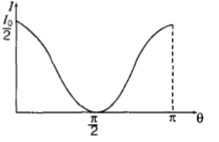Using {tex}I _ { 2 } = I _ { 1 } \cos ^ { 2 } \theta{/tex}
2. Given
I1 = intensity of light transmitted by the polaroid P1
I2 = intensity of light transmitted by the polaroid P2
I3 = intensity of light transmitted by the polaroid P3
According to Malus law of polarisation,
{tex}I _ { 3 } = I _ { 1 } \cos ^ { 2 } \beta{/tex} ….. (i)
{tex}I _ { 2 } = I _ { 3 } \cos ^ { 2 } ( \theta – \beta ){/tex} …… (ii)
According to question
I2 = I3
Substituting the value of I2 and I3 from Eq. (i) and Eq. (ii) in the above condition, we get
{tex}I _ { 3 } \cos ^ { 2 } ( \theta – \beta ) = I _ { 1 } \cos ^ { 2 } \beta{/tex} …..(iii)
Substituting the value of I3 from equation (i) into equation (iii)
{tex}I _ { 1 } \cos ^ { 2 } \beta \cos ^ { 2 } ( \theta – \beta ) = I _ { 1 } \cos ^ { 2 } \beta{/tex}
{tex}\Rightarrow \cos ^ { 2 } ( \theta – \beta ) = 1{/tex}
{tex}\Rightarrow ( \theta – \beta ) = \cos ^ { – 1 } ( 1 ){/tex}
{tex}\Rightarrow ( \theta – \beta ) = 0{/tex}
{tex}\theta = \beta{/tex} ……. (iv)
According to question I1 = I2
Substituting the value of I2 from Eq. (ii),
{tex}I _ { 1 } = I _ { 3 } \cos ^ { 2 } ( \theta – \beta ){/tex} …..(v)
Substituting the value of I3 from Eq. (i) into eq. (v)
{tex}I _ { 1 } = I _ { 1 } \cos ^ { 2 } \beta \cos ^ { 2 } ( \theta – \beta ){/tex}
or {tex}\cos ^ { 2 } \beta = 1{/tex} [From Eq. (iv), {tex}\theta = \beta{/tex}] {tex}\beta = 0 ^ { \circ } \text { or } \pi{/tex}
9. Angular width is given by{tex}\theta = \frac { \lambda } { d } \text { or } d = \frac { \lambda } { \theta }{/tex}
1. According to the question , {tex}\lambda = 600nm = 6 \times {10^{ – 7}}m{/tex}
{tex}\theta = \frac { 0.1 \pi } { 180 } \mathrm { rad } = \frac { \pi } { 1800 } \mathrm { rad }{/tex}
{tex} d = \frac { \lambda } { \theta }{/tex}
{tex}\therefore d = \frac { 6 \times 10 ^ { – 7 } \times 1800 } { \pi } = 344 \times 10 ^ { – 4 } \mathrm { m }{/tex}
2. The frequency of a light depends on its source only.
So, the frequencies of reflected and refracted light will be same as that of incident light.
Reflected light is in the same medium (air).
so its wavelength remains same as {tex}500\mathop A\limits^o {/tex} .
we know that {tex}\nu=\frac{c}{\lambda}{/tex}
{tex}= \frac {3 \times 10^8}{5000 \times 10^{-10}}{/tex}
{tex}=6 \times 10^{18}{ Hz}.{/tex}
This is the required frequency of both refracted and reflected light.
we know that {tex}\mu = \frac{speed \ of \ light \ in \ air}{speed \ of \ light \ in \ water}{/tex}
{tex}\frac {4}{3}= \frac {3\times 10^8}{v}{/tex}
{tex}v = 2.25 \times 10^8 m/s{/tex}
speed of light in water = {tex}2.25 \times10^8 m/s{/tex}
Wavelength of refracted light is given by{tex}\lambda { ‘} ={v\over \nu} = 0.375 \times 10^{-6} m{/tex}
So, wavelength of refracted wave will be decreased.

## Chapter Wise Extra Questions of Class 12 Physics Part I & Part II## Test Generator

Create Papers with your Name & Logo